# SAS/ETS Examples

## Estimating an Almost Ideal Demand System Model

Contents | SAS Program

# Overview

The Almost Ideal Demand System (AIDS) model of Deaton and Muellbauer (1980b) has enjoyed great popularity in applied demand analysis. Starting from a specific cost function, the AIDS model gives the share equations in an n-good system as
where wi is the share associated with the ith good,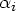is the constant coefficient in the ith share equation,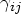is the slope coefficient associated with the jth good in the ith share equation, pj is the price on the jth good. X is the total expenditure on the system of goods given by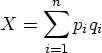in which qi is the quantity demanded for the ith good. P is the price index defined by
in the nonlinear AIDS model. Deaton and Muellbauer (1980a) also suggested a linear approximation of the nonlinear AIDS model by specifying a linear price index given by
that gives rise to the linear approximate AIDS (LA-AIDS) model. In practice, the LA-AIDS model is more frequently estimated than the nonlinear AIDS model.

Conservation implies the following restrictions on the parameters in the nonlinear AIDS model:
Homogeneity is satisfied if and only if, for all i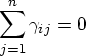and symmetry is satisfied if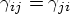One advantage of the AIDS model is that the homogeneity and symmetry restrictions are easily imposed and tested.

# Analysis

In this example, you can estimate a nonlinear AIDS model and LA-AIDS model using U.S. meat products data. The data consists of aggregate quarterly retail price and per capita consumption for four meat product categories including beef, pork, chicken, and turkey. The data period covers the first quarter of 1974 to the last quarter of 1999. The data were obtained from various USDA sources listed in the references. More detailed description of the data construction may be found in Piggott (1997).

To incorporate seasonality and trend in the U.S. meat consumption data, you augment the AIDS model with trigonometric variables and a time trend variable. Thus the share equations to be estimated are expressed as follows:
where aci and asi represent parameters on the trigonometric variables, and ati is the parameter on the time trend variable. Since the budget shares sum up to 1, these parameters should satisfy
The following is a portion of the code used to create the data set. A second set of SAS statements is used to create the time trend variable and the seasonal variables. Total expenditures, X, and shares for each good are also computed.

   data aids_;
input year qtr pop beef_q pork_q chick_q turkey_q beef_p pork_p chick_p
turkey_p cpi pc_exp;
date = yyq(year,qtr);
format date yyq6.;

... more datalines ...

;
run;


   data aids;
set aids_;
if year < 1975 then delete;
t = _n_ ;
co1 = cos(1/2*3.14159*t);
co2 = cos(2/2*3.14159*t);

si1 = sin(1/2*3.14159*t);
si2 = sin(2/2*3.14159*t);

x = beef_p*beef_q + pork_p*pork_q + chick_p*chick_q
+ turkey_p*turkey_q;

w_beef = beef_p*beef_q/x;
w_pork = pork_p*pork_q/x;
w_chick = chick_p*chick_q/x;
w_turkey = turkey_p*turkey_q/x;
run;


The plot of the budget shares found in Figure 1.

   proc gplot data=aids;
plot w_beef*date w_pork*date w_chick*date w_turkey*date/
overlay cframe=ligr haxis=axis1 vaxis=axis2;
title 'Budget Shares Plots';
footnote1 c=blue '  *     beef   '
c=red  '  .     pork  '
c=green '  o    chicken  '
c=black '  +    turkey   ';
symbol1 c=blue i=join v=star;
symbol2 c=red  i=join v=dot;
symbol3 c=green i = join v = circle ;
symbol4 c=black i = join v = plus ;
axis1  label=('Time') ;
axis2  label=(angle=90 'Budget Share');
run;
quit;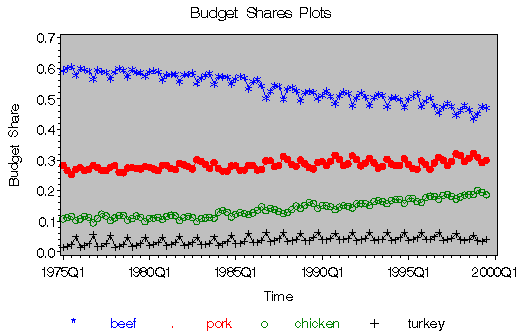Figure 1: Budget Shares Plots

Price and quantity variables in the data set are labeled in an obvious manner. Three variable names in the DATA step require some explanation. The variable named pop contains the U.S. population, cpi contains the consumer price index for all goods, and pc_exp is the per capita nominal personal consumption expenditures on all goods.

To obtain mean-scaled price data, you use the MEANS procedure. The means of each of the price variables are written to the OUT= data set as the b_m, p_m, c_m, and t_m variables. The original price variables are then divided by the mean variables in a subsequent DATA step.

   data aids ;
if _n_ = 1 then set pm ;
set aids ;

pb_ = (beef_p/b_m);
pp_ = (pork_p/p_m);
pc_ = (chick_p/c_m);
pt_ = (turkey_p/t_m);

lpb = log(pb_);
lpp = log(pp_);
lpc = log(pc_);
lpt = log(pt_);

lx = log(x);

/* Specify the linear price index for the LA-AIDS model */
lp0 = sum(w_beef*lpb + w_pork*lpp + w_chick*lpc + w_turkey*lpt);
lxp = lx-lp0;
run;


The LA-AIDS model is estimated using the SYSLIN procedure. The ITSUR option specifies the iterated seemingly unrelated regression as the estimation method. The equations to be fit are then specified. Since budget shares sum to 1 in the system, one of the share equations is deleted to deal with the singularity problem. In this example, you eliminate the share equation for turkey. Whichever one is eliminated should not have any effect on the results. The parameters associated with the share equation that is deleted can be recovered through the parameter restrictions implied by the homogeneity, symmetry, and conservation properties. The parameter restrictions can be imposed through the SRESTRICT statement.

   proc syslin itsur data = aids outest = fin1;
b: model w_beef = lpb lpp lpc lpt lxp co1 si1 t ;
p: model w_pork = lpb lpp lpc lpt lxp co1 si1 t ;
c: model w_chick = lpb lpp lpc lpt lxp co1 si1 t ;

srestrict
/*symmetry restrictions */
b.lpp = p.lpb ,
b.lpc = c.lpb ,
p.lpc = c.lpp ,
/* homogeneity restrictions*/
b.lpb + b.lpp + b.lpc + b.lpt = 0 ,
p.lpb + p.lpp + p.lpc + p.lpt = 0 ,
c.lpb + c.lpp + c.lpc + c.lpt = 0;
run;


The parameter estimates in the beef equation are given in Figure 2.

 Parameter Estimates - Beef

Variable DF Estimate StdErr tValue Probt
Intercept 1 0.397899 0.400708 0.99 0.3234
lpb 1 0.041175 0.012686 3.25 0.0016
lpp 1 -0.00407 0.008127 -0.50 0.6181
lpc 1 -0.04926 0.00541 -9.10 <.0001
lpt 1 0.012151 0.009613 1.26 0.2095
lxp 1 0.023424 0.044316 0.53 0.5984
co1 1 -0.01631 0.001631 -10.00 <.0001
si1 1 -0.00228 0.001571 -1.45 0.1497
t 1 -0.00148 0.000049 -30.45 <.0001

Figure 2: Parameter Estimates for Beef

The parameter estimates in the pork equation are given in Figure 3

 Parameter Estimates - Pork

Variable DF Estimate StdErr tValue Probt
Intercept 1 0.240957 0.28773 0.84 0.4046
lpb 1 -0.00407 0.008127 -0.50 0.6181
lpp 1 0.044733 0.008811 5.08 <.0001
lpc 1 -0.03074 0.006022 -5.10 <.0001
lpt 1 -0.00993 0.007543 -1.32 0.1915
lxp 1 0.003386 0.031819 0.11 0.9155
co1 1 0.009611 0.001187 8.10 <.0001
si1 1 0.006454 0.001137 5.68 <.0001
t 1 0.000278 0.000036 7.70 <.0001

Figure 3: Parameter Estimates for Pork

The parameter estimates in the chicken equation are given in Figure 4

 Parameter Estimates - Chicken

Variable DF Estimate StdErr tValue Probt
Intercept 1 0.579604 0.17659 3.28 0.0015
lpb 1 -0.04926 0.00541 -9.10 <.0001
lpp 1 -0.03074 0.006022 -5.10 <.0001
lpc 1 0.101387 0.007607 13.33 <.0001
lpt 1 -0.02139 0.006996 -3.06 0.0029
lxp 1 -0.05355 0.019548 -2.74 0.0074
co1 1 -0.00567 0.0007 -8.10 <.0001
si1 1 -0.00084 0.000664 -1.26 0.2114
t 1 0.000893 0.000026 33.86 <.0001

Figure 4: Parameter Estimates for Chicken

The parameter restrictions estimates are reported in Figure 5:

 Parameter Restrictions Estimates

Variable DF Estimate StdErr tValue Probt
RESTRICT -1 -233.279 65.95364 -3.54 0.0003
RESTRICT -1 -130.174 75.45235 -1.73 0.0845
RESTRICT -1 -14.1833 95.49745 -0.15 0.8829
RESTRICT -1 158.8543 73.2354 2.17 0.0293
RESTRICT -1 -36.2377 88.88858 -0.41 0.6859
RESTRICT -1 -301.062 110.8651 -2.72 0.0059

Figure 5: Parameter Restrictions Estimates

For the nonlinear AIDS model, you estimate the model using the MODEL procedure. Homogeneity restrictions can easily be imposed using the RESTRICT statement. The symmetry restrictions can be imposed by using the same parameter name for the corresponding variables. The nonlinear price index needs to be specified as part of the model program. The FIT statement estimates model parameters by fitting the specified equations to the data. The ITSUR option in the FIT statement specifies the estimation method as the iterated seemingly unrelated regression method. The parameters to be estimated are specified in the PARMS statement.

   /* Full Nonlinear AIDS Model */
proc model data=aids;

/* imposing homogeneity and symmetry and adding-up restrictions */
restrict gbb + gbp + gbc + gbt = 0 ,
gbp + gpp + gpc + gpt = 0 ,
gbc + gpc + gcc + gct = 0 ,
gbt + gpt + gct + gtt = 0 ,
ab + ap + ac + at = 1 ;

/*non-linear price index*/
a0 = 0; /* restrict the constant term in the nonlinear price index to be zero */
p = a0 + ab*lpb + ap*lpp + ac*lpc + at*lpt +
.5*(gbb*lpb*lpb + gbp*lpb*lpp + gbc*lpb*lpc + gbt*lpb*lpt +
gbp*lpp*lpb + gpp*lpp*lpp + gpc*lpp*lpc + gpt*lpp*lpt +
gbc*lpc*lpb + gpc*lpc*lpp + gcc*lpc*lpc + gct*lpc*lpt +
gbt*lpt*lpb + gpt*lpt*lpp + gct*lpt*lpc + gtt*lpt*lpt );

/*share equation*/
w_beef = ab + gbb*lpb + gbp*lpp + gbc*lpc + gbt*lpt + bb*(lx-p)
+ abco1*co1 + absi1*si1 + ab_t*t ;
w_pork = ap + gbp*lpb + gpp*lpp + gpc*lpc + gpt*lpt + bp*(lx-p)
+ apco1*co1 + apsi1*si1 + ap_t*t ;
w_chick = ac + gbc*lpb + gpc*lpp + gcc*lpc + gct*lpt + bc*(lx-p)
+ acco1*co1 + acsi1*si1 + ac_t*t ;

fit w_beef w_pork w_chick / itsur nestit outs=rest estdata=fin0 outest=fin2
out = resid2 converge = .00001  maxit = 1000 ;
parms ab bb gbb gbp gbc gbt abco1 absi1 ab_t
ap bp     gpp gpc gpt apco1 apsi1 ap_t
ac bc         gcc gct acco1 acsi1 ac_t
at                gtt ;

run;
quit;


Note that in the nonlinear AIDS model, two additional parameters are involved, namely, gtt and at. These parameters appear in the nonlinear price index specification. The parameter estimates for the nonlinear AIDS model are given in Figure 6

The MODEL Procedure

Nonlinear ITSUR Parameter Estimates
Parameter Estimate Approx Std Err t Value Approx
Pr > |t|
Label
gbb 0.037238 0.0176 2.11 0.0373
gbp -0.00538 0.00834 -0.65 0.5203
gbc -0.03931 0.0232 -1.69 0.0938
gbt 0.007449 0.0114 0.66 0.5137
gpp 0.043685 0.0111 3.94 0.0002
gpc -0.02431 0.0167 -1.46 0.1489
gpt -0.014 0.0112 -1.25 0.2140
gcc 0.071597 0.0195 3.67 0.0004
gct -0.00798 0.0191 -0.42 0.6766
gtt 0.014533 0.0215 0.68 0.5013
ab 0.377488 0.3819 0.99 0.3255
ap 0.158295 0.2667 0.59 0.5543
ac 0.632779 0.1670 3.79 0.0003
at -0.16856 0.2827 -0.60 0.5525
bb 0.02569 0.0423 0.61 0.5448
abco1 -0.01637 0.00162 -10.11 <.0001
absi1 -0.00224 0.00157 -1.43 0.1552
ab_t -0.00148 0.000049 -30.07 <.0001
bp 0.01255 0.0295 0.43 0.6716
apco1 0.00945 0.00117 8.10 <.0001
apsi1 0.006587 0.00112 5.86 <.0001
ap_t 0.000275 0.000036 7.69 <.0001
bc -0.05947 0.0185 -3.22 0.0018
acco1 -0.00554 0.000687 -8.06 <.0001
acsi1 -0.00094 0.000653 -1.44 0.1523
ac_t 0.000899 0.000026 34.15 <.0001
Restrict0 -170.721 74.3756 -2.30 0.0209 gbb + gbp + gbc + gbt = 0
Restrict1 13.41517 92.9286 0.14 0.8861 gbp + gpp + gpc + gpt = 0
Restrict2 282.4448 115.5 2.44 0.0137 gbc + gpc + gcc + gct = 0
Restrict3 -1.55944 0.7147 -2.18 0.0283 gbt + gpt + gct + gtt = 0
Restrict4 21.02431 6.7622 3.11 0.0015 ab + ap + ac + at = 1

Figure 6: Parameter Estimates for the Nonlinear AIDS Model

Figure 7 illustrates the residuals obtained for the beef, pork, and chicken equations.

   proc gplot data = resid2;

plot w_beef*date w_pork*date w_chick*date / overlay cframe=ligr
haxis=axis1 vaxis=axis2 vref=0;
title 'Residual Plots';

footnote1 c=blue '  *     beef   '
c=red  '  .     pork  '
c=green '  o    chicken  ';

symbol1 c=blue i=join v=star;
symbol2 c=red  i=join v=dot;
symbol3 c=green i = join v = circle ;

axis1  label=('Time') ;
axis2  label=(angle=90 'Residuals');
run;
quit;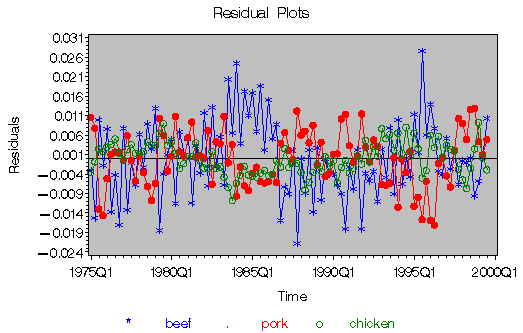Figure 7: Beef,Pork,and Chicken Residuals

The estimated parameters are stored in data sets and will be used in calculating price and income elasticities in the example "Calculating Elasticities in an Almost Ideal Demand System."

# Acknowledgment

The SAS program used in this example is based on code provided by Dr. Barry Goodwin.

# References

Deaton, A., and Muellbauer, J. (1980a), "An Almost Ideal Demand System," American Economic Review, 70, 312-336.

Deaton, A., and Muellbauer, J. (1980b), Economics and Consumer Behavior, Cambridge, England: Cambridge University Press.

Piggott, N. (1997), The Benefits and Costs of Generic Advertising of Agricultural Commodities, Ph.D. dissertation, University of California, Davis.

SAS Institute Inc. (1999), SAS/ETS User's Guide, Version 8, Cary, NC: SAS Institute Inc.

USDA-ERS Electronic Data Archive, Red Meats Yearbook, housed at Cornell University's Mann Library, [http://usda.mannlib.cornell.edu/], accessed 25 September 2001.

USDA-ERS Electronic Data Archive, Poultry Yearbook, housed at Cornell University's Mann Library, [http://usda.mannlib.cornell.edu/], accessed 25 September 2001.